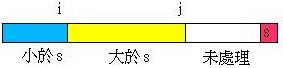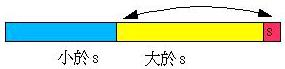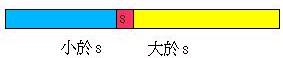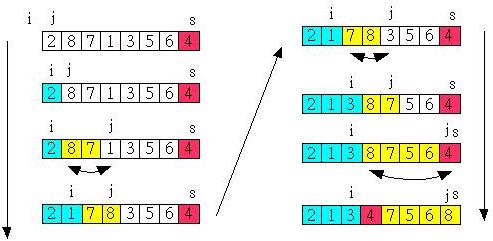## 二、快速排序实现

### 2.1 快速排序基础版

（注意：*表示要交换的数，[]表示轴）

• 　24　76*　11　45　64　21　69　19　36*
• 　24　36　11　45*　64　21　69　19*　76
• 　24　36　11　19　64*　21*　69　45　76
• 　24　36　11　19　21　64　69　45　76
• 21　24　36　11　19　　64　69　45　76

1. 令索引 i 从数列左方往右方找，直到找到大于 s 的数
2. 令索引 j 从数列右方往左方找，直到找到小于 s 的数
3. 如果 i >= j，则离开回圈
4. 如果 i < j，则交换索引i与j两处的值
5. 将左侧的轴与 j 进行交换
6. 对轴左边进行递回
7. 对轴右边进行递回

``````package main

import (
"fmt"
)

const MAX = 10

var sortArray = []int{41, 24, 76, 11, 45, 64, 21, 69, 19, 36}

func main() {

fmt.Println("before sort：")
show()

quickSort(sortArray, 0, MAX-1)

fmt.Println("after sort:")
show()

}

// quickSort
func quickSort(sortArray []int, left, right int) {

if left < right {
key := sortArray[left]
i := left
j := right

for {
for i+1 < MAX {
i++
if key <= sortArray[i] {
break
}
}

for j-1 >= 0 {
if key >= sortArray[j] {
break
}
j--
}

if i >= j {
break
}

swap(i, j)
}

sortArray[left] = sortArray[j]
sortArray[j] = key
show()

quickSort(sortArray, left, j-1)
quickSort(sortArray, j+1, right)
}

}

// Swap the position of a and b
func swap(a, b int) {
sortArray[a], sortArray[b] = sortArray[b], sortArray[a]
}

// foreach
func show() {
for _, value := range sortArray {
fmt.Printf("%d\t", value)
}
}``````

### 2.2 快速排序升级版

41　24　76　11　45　64　21　69　19　36

• 41　24　76*　11　　64　21　69　19　*36
• 41　24　36　11　45*　64　21　69　19*　76
• 41　24　36　11　19　64*　21*　69　45　76
• [41　24　36　11　19　21]　[64　69　45　76]

``````package main

import (
"fmt"
)

const MAX = 10

var sortArray = []int{41, 24, 76, 11, 45, 64, 21, 69, 19, 36}

func main() {

fmt.Println("before sort：")
show()

quickSort(sortArray, 0, MAX-1)

fmt.Println("after sort:")
show()

}

func quickSort(sortArray []int, left, right int) {
if left < right {
key := sortArray[(left+right)/2]
i := left
j := right

for {
for sortArray[i] < key {
i++
}
for sortArray[j] > key {
j--
}
if i >= j {
break
}
swap(i, j)
}

quickSort(sortArray, left, i-1)
quickSort(sortArray, j+1, right)
}
}

// Swap the position of a and b
func swap(a, b int) {
sortArray[a], sortArray[b] = sortArray[b], sortArray[a]
}

// foreach
func show() {
for _, value := range sortArray {
fmt.Printf("%d\t", value)
}
}``````

### 2.3 快速排序最终版``````QUICKSORT(A, p, r)
if p < r
then q <- PARTITION(A, p, r)
QUICKSORT(A, p, q-1)
QUICKSORT(A, q+1, r)
end QUICKSORT

PARTITION(A, p, r)
x <- A[r]
i <- p-1
for j <- p to r-1
do if A[j] <= x
then  i <- i+1
exchange A[i]<->A[j]
exchange A[i+1]<->A[r]
return i+1
end PARTITION````````````package main

import (
"fmt"
)

const MAX = 10

var sortArray = []int{41, 24, 76, 11, 45, 64, 21, 69, 19, 36}

func main() {

fmt.Println("before sort：")
show()

quickSort(sortArray, 0, MAX-1)

fmt.Println("after sort:")
show()

}

func quickSort(sortArray []int, left, right int) {
if left < right {
pos := partition(sortArray, left, right)
quickSort(sortArray, left, pos-1)
quickSort(sortArray, pos+1, right)
}
}

func partition(sortArray []int, left, right int) int {
key := sortArray[right]
i := left - 1

for j := left; j < right; j++ {
if sortArray[j] <= key {
i++
swap(i, j)
}
}

swap(i+1, right)

return i + 1
}

// Swap the position of a and b
func swap(a, b int) {
sortArray[a], sortArray[b] = sortArray[b], sortArray[a]
}

// foreach
func show() {
for _, value := range sortArray {
fmt.Printf("%d\t", value)
}
}``````